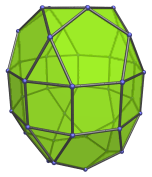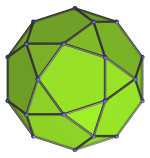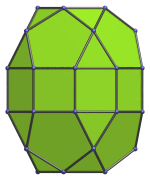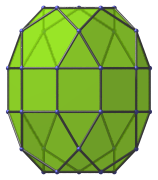# The Elongated Pentagonal Orthobirotunda

The elongated pentagonal orthobirotunda is the 42nd Johnson solid (J42). It has 40 vertices, 80 edges, and 42 faces (20 equilateral triangles, 10 squares, 12 pentagons).The elongated pentagonal orthobirotunda can be constructed by attaching two pentagonal rotundae to a decagonal prism, or equivalently, inserting a decagonal prism between the two halves of a pentagonal orthobirotunda (J34). The ortho- in the name refers to how the top and bottom pentagons are aligned with each other. If they are rotated with respect to each other instead, the elongated pentagonal gyrobirotunda (J43) is produced instead.

## Projections

Here are some views of the elongated pentagonal orthobirotunda from various angles:

Projection DescriptionTop view.Front view.Side view.

## Coordinates

The Cartesian coordinates of the elongated pentagonal orthobirotunda with edge length 2 are:

• (−√((10+2√5)/5), 0, ±(1+√((20+8√5)/5)))
• (−√((5−√5)/10), ±φ, ±(1+√((20+8√5)/5)))
• ( √((5+2√5)/5), ±1, ±(1+√((20+8√5)/5)))
• ( √((20+8√5)/5), 0, ±(1+√((10+2√5)/5)))
• (−√((25+11√5)/10), ±φ, ±(1+√((10+2√5)/5)))
• ( √((5+√5)/10), ±φ2, ±(1+√((10+2√5)/5)))
• (±√(3+4φ), ±1, ±1)
• (±√(2+φ), ±φ2, ±1)
• (0, ±2φ, ±1)

where φ=(1+√5)/2 is the Golden Ratio.

Last updated 17 May 2018.